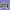Australia's Leading Digital Marketing Experts. T. 1300 235 433  |  Aggregation Enquires Welcome

# Add st, nd, rd, th to a Number with PHP

This simple function will add st, nd, rd or th to a cardinal number (the suffix acts as an ordinal indicator). There are plenty of these functions floating around but only a few take the subtle variances into consideration that can screw up the result.

If you're using PHP 5 >= 5.3.0 and you have PECL intl >= 1.0.0 installed, consider PHP's class.numberformatter.

### The PHP Function

1
<?php
2
/*
3
Add st, nd, rd, th to a Number with PHP
4
5
*/
6

7
function beliefmedia_ordinal(\$cardinal) {
8
\$test_c = abs(\$cardinal) % 10;
9
\$extension = ((abs(\$cardinal) %100 < 21 && abs(\$cardinal) %100 > 4) ? 'th' : ((\$test_c < 4) ? (\$test_c < 3) ? (\$test_c < 2) ? (\$test_c < 1) ? 'th' : 'st' : 'nd' : 'rd' : 'th'));
10
return \$cardinal . \$extension;
11
}

Usage

To append the appropriate suffix to the ordinal number, use the following:

1
echo beliefmedia_ordinal(14);

The resulting cardinal number will print as 14th.

If you would like to test the code, use the following loop that'll print the 1st through to the 99th.

1
<?php
2
for(\$i=1; \$i<100; \$i++) {
3
echo beliefmedia_ordinal(\$i) . '';
4
}